##### Generating Graphs

Many graphs consistently prove interesting, in the sense that they are models of important binary relations or have unique graph theoretic properties. Often, these graphs can be parameterized, such as the complete graph on $n$ vertices $K_n$, giving a concise notation for expressing an infinite class of graphs. We start off with several operations that act on graphs to give different graphs and which, together with our parameterized graphs, give us the means to construct essentially any interesting graph.

The union of two connected graphs has two connected components.

`ShowGraph[ GraphUnion[ CompleteGraph, CompleteGraph[5,5] ] ];`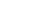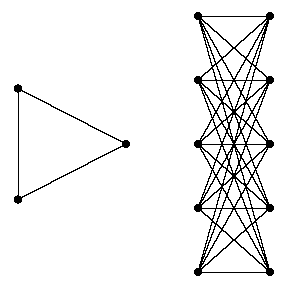Graph products can be very interesting. The embedding of a product has been designed to show off its structure, and is formed by shrinking the first graph and translating it to the position of each vertex in the second graph.

`ShowGraph[ GraphProduct[ CompleteGraph, CompleteGraph ] ];`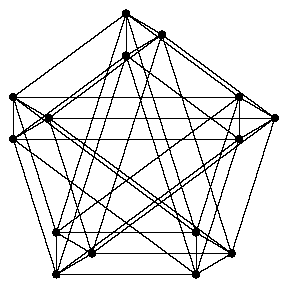The line graph $L\left(G\right)$ of a graph $G$ has a vertex of $L\left(G\right)$ associated with each edge of $G$ and an edge of $L\left(G\right)$ if and only if the two edges of $G$ share a common vertex.

`ShowGraph[ LineGraph[CompleteGraph] ];`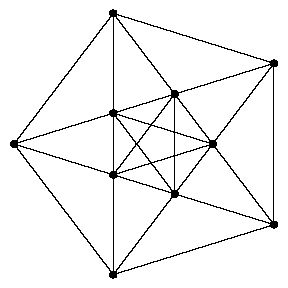Circulants are graphs whose adjacency matrix can be constructed by rotating a vector $n$ times, and include complete graphs and cycles as special cases. Even random circulant graphs have an interesting, regular structure.

`ShowGraph[ CirculantGraph[21, RandomKSubset[Range,3]]];`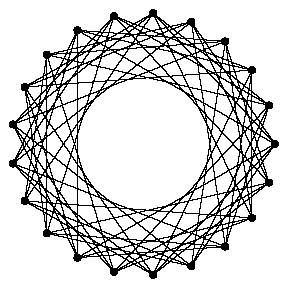This random graph can be expected to have half the number of edges of a complete graph, even though all labeled graphs occur with equal probability.

`ShowGraph[ RandomGraph[20,0.5] ];`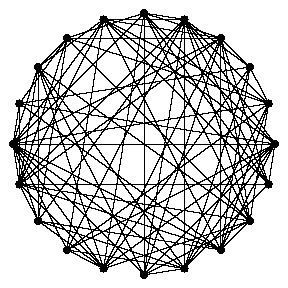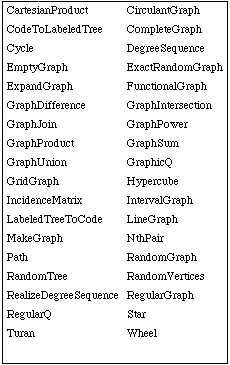Combinatorica functions for generating graphs.